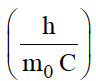The Compton wavelength for an electron is, calculate $∆\lambda$(1- cos θ) for a scattering angle  θ = $\mathrm{\pi }$/3. What is the maximum value for $∆\lambda$ ?

(A) 0.024 Å, 0.048 Å

(B) 0.012 Å, 0.048 Å

(C)0.012 Å, 0.024 Å

(D) 0.006 Å, 0.012 Å

Concept Questions :-

High Yielding Test Series + Question Bank - NEET 2020

Difficulty Level:

The minimum value of spin multiplicity possible, when $\mathcal{l}$=3, are

(A) 2

(B) 1

(C) 0

(D) 3

Concept Questions :-

Quantum Numbers
High Yielding Test Series + Question Bank - NEET 2020

Difficulty Level:

Positronium is an unstable complex of electron and positron, both of the same mass, but opposite charge. Apply Bohr's theory of hydrogen atom to this system and obtain the energy required to separate the electron from the positron.

(ionization energy of the ground state hydrogen atom= 13.62eV.) Express the result in KJmol-1

(A) 550 KJmol-1

(B) 657 KJmol-1

(C) 851 KJmol-1

(D) 720 KJmol-1

Concept Questions :-

Bohr
High Yielding Test Series + Question Bank - NEET 2020

Difficulty Level:

The total spin resulting from a d7 configuration is -

(A) 3/2

(B) 1/2

(C) 2

(D) 1

Concept Questions :-

Quantum Numbers
High Yielding Test Series + Question Bank - NEET 2020

Difficulty Level:

Supposing the energy of fourth shell for hydrogen atom is -50a.u. (Arbitrary unit). What would be its ionization potential-

(A) 50

(B) 800

(C) 15.4

(D) 20.8

Concept Questions :-

Bohr
High Yielding Test Series + Question Bank - NEET 2020

Difficulty Level:

An element with atomic mass Z consists of two isotopes of mass number Z-1 and Z + 2. The percentage abundance of the heavier isotope is -

(A) 0.25

(B)33.3

(C)66.6

(D)75

Concept Questions :-

introduction
High Yielding Test Series + Question Bank - NEET 2020

Difficulty Level:

Two isotopes of Boron are found in the nature with atomic weights 10.01(I) and 11.01(II). The atomic weight of natural Boron is 10.81. The percentage of (I) and (II) isotopes in it are respectively-

(A) 20 and 80

(B) 10 and 90

(C) 15 and 75

(D) 30 and 70

Concept Questions :-

introduction
High Yielding Test Series + Question Bank - NEET 2020

Difficulty Level:

Calculate the wave-number  of lines having the frequency of 5 x 1016 cycles per sec.

(A) 1.666 x 108m-1

(B) 0.666 x 108m-1

(C) 4.126 x 106m-1

(D) 3.133 x 104m-1

Concept Questions :-

High Yielding Test Series + Question Bank - NEET 2020

Difficulty Level:

Which of the following set of quantum number is not valid

(A) n = 1 , $\mathcal{l}$ = 2

(B) n=2, m = 1

(C) n =3, $\mathcal{l}$ = 0

(D) n = 4, $\mathcal{l}$ = 2

Concept Questions :-

Quantum Numbers
High Yielding Test Series + Question Bank - NEET 2020

Difficulty Level:

For particles having same kinetic energy, the de Broglie wavelength is-

(A) Directly proportional to its velocity

(B) Inversely proportional to its velocity

(C) Independent of velocity and mass

(D) Unpredictable

Concept Questions :-

de Broglie Equation